Documentation

## Release 14 V2X Sidelink PSCCH and PSSCH Throughput

This example demonstrates how to measure the Physical Sidelink Shared Channel (PSSCH) and Physical Sidelink Control Channel (PSCCH) throughput performance in frequency-selective fading and Additive White Gaussian Noise (AWGN) using LTE Toolbox™.

### Introduction

3GPP Release 14 introduced the support for LTE V2X (Vehicle-to-everything) to enable connected vehicular services with the aim of providing a safer, cleaner, faster and more efficient transportation. V2X offers several modes of operation including Vehicle-to-Vehicle (V2V), Vehicle-to-Infrastructure (V2I) and Vehicle-to-Pedestrian (V2P) direct communication without necessarily relying on network involvement for scheduling. Some of the distinguishing aspects of V2X over the Release 12 device-to-device sidelink are:

• Low latency and high reliability requirements

• Large Doppler shift due to high relative speeds

• Very large number of nodes and high node densities

• Challenges to synchronization especially when out-of-coverage

This example measures Release 14 V2X sidelink shared and control channel throughput for a number of SNR points. For information on how to model Release 12 sidelink device-to-device (D2D) interface (aimed primarily at allowing LTE to support public safety communication systems), see the Release 14 V2X Sidelink PSCCH and PSSCH Throughput example.

Operating on a subframe by subframe basis for each SNR point, the following steps are performed for the throughput and BLER calculation:

• A resource grid populated with PSCCH and/or PSSCH is generated and OFDM modulated to create the baseband waveform to transmit

• This waveform is passed through a noisy fading channel

• Receiver operations (SC-FDMA demodulation, channel estimation, and equalization) are performed

• The equalized symbols are decoded to obtain the block CRC

• The performance of the PSCCH and/or PSSCH is determined using the block CRC result at the output of the channel decoder

### Simulation Configuration

The example is executed for a simulation length of 4 frames for a number of SNR points. A larger number of `NFrames` should be used to produce meaningful throughput results. `SNRIn` can be an array of values or a scalar.

```% Set the number of frames to simulate NFrames = 4; % Set a range of SNR points to cover both high and low BLER operating % conditions SNRIn = [-5 0 5]; ```

### Transmission Configuration

A set of top-level parameters is initially specified, these include the bandwidth, the cyclic prefix, the modulation scheme and the allocated set of resource blocks. The baseline configuration is taken from the "Reference measurement channel for transmitter characteristics" as defined by TS 36.101 Table A.8.3-1 [ 2 ]. To simulate a more realistic V2X transmission, multiple HARQ processes and HARQ retransmissions have been introduced in this example. The data rate is defined in terms of the subframe gap `sfGap` and transmission time interval `tti`.

```sfGap = 8; % Time in subframes between initial and retransmission tti = 4; % Time in subframes between different HARQ processes if tti > sfGap error('tti cannot be greater than sfGap'); end % Number of allocated resource blocks (NPRB) and Transport Block Size (TBS) % as given by TS 36.101 Table A.8.3-1. Note that TBS must be chosen % according to TS 36.213 section 14.1.1 and NPRB according to TS 36.213 % section 14.1.1.4C. If an invalid TBS/NPRB is specified, the transmitted % ModCoding value in the SCI message would be incorrect and this could % result in the SL-SCH decoding to fail. An invalid NPRB could also result % in an SCI message and corresponding PSSCH transmission where the % allocated resources are different from the NPRB specified here. NPRB = 48; % Number of allocated resource blocks (NPRB) TBS = 3496; % The transport block size % Define the starting RB for the resource allocations. The initial and % subsequent transmission can have different allocations rbStart = [0 0]; % Define the UE configuration parameters ueConfig = struct('SidelinkMode','V2X');% Release 14 V2X mode ueConfig.NSLRB = 50; % 10MHz bandwidth ueConfig.DuplexMode = 'FDD'; % Duplex mode ueConfig.CyclicPrefixSL = 'Normal'; % Cyclic prefix length ueConfig.Modulation = 'QPSK'; % Symbol modulation ('QPSK','16QAM') ```

### Propagation Channel Configuration

The structure `channel` contains the channel model configuration parameters.

```channel = struct; % Channel config structure channel.Seed = 6; % Channel seed channel.NRxAnts = 2; % Number of receive antennas channel.DelayProfile ='EVA'; % Delay profile channel.DopplerFreq = 500; % Doppler frequency in Hz channel.MIMOCorrelation = 'Low'; % Multi-antenna correlation channel.NTerms = 16; % Oscillators used in fading model channel.ModelType = 'GMEDS'; % Rayleigh fading model type channel.InitPhase = 'Random'; % Random initial phases channel.NormalizePathGains = 'On'; % Normalize delay profile power channel.NormalizeTxAnts = 'On'; % Normalize for transmit antennas % The channel sampling rate depends on the FFT size used in the OFDM % modulator. This can be obtained using the function lteSLSCFDMAInfo. ofdmInfo = lteSLSCFDMAInfo(ueConfig); channel.SamplingRate = ofdmInfo.SamplingRate; ```

### Channel Estimator Configuration

The variable `perfectChanEstimator` controls channel estimator behavior. Valid values are `true` or `false`. When set to `true`, a perfect channel response is used as estimate, otherwise an imperfect estimate based on the values of received DM-RS signals is obtained. If `perfectChanEstimator` is set to false a configuration structure `cec` is needed to parameterize the channel estimator.

```% Perfect channel estimator flag perfectChanEstimator = false; % Define the practical channel estimator configuration structure. Note that % depending on the channel delay profile and Doppler frequency, the % operating SNR, PSSCH modulation order and the number of allocated % resource blocks, different channel estimation parameters may give better % performance. cec = struct; % Channel estimation config structure cec.PilotAverage = 'UserDefined'; % Type of pilot symbol averaging cec.FreqWindow = 27; % Frequency window size cec.TimeWindow = 1; % Time window size cec.InterpType = 'Cubic'; % 2D interpolation type ```

### Configure Throughput Measurements

The logical variables `measureBLERForSCI` and `measureTputForSLSCH` allow the throughput measurements and all related receiver processing to be disabled for the SCI and SL-SCH respectively. This allows the simulation to be configured to measure throughput for only one of the channels. Disabling the receiver processing for the other channel improves the execution speed.

```measureBLERForSCI = true; measureTputForSLSCH = true; ```

### Select Receiver Behavior When SCI Decoding Fails

The logical variable `sciAssumed` controls the simulation behavior in terms of the effect the control channel decoding has on the shared channel decoding. If `sciAssumed` is true, the receiver will assume that the SCI message was correctly decoded when attempting shared channel reception. This allows the performance of the shared channel reception to be measured independently from the performance of the control channel reception. If `sciAssumed` is false, a control channel decoding failure implies a shared channel decoding failure. Note that if the throughput measurement for the SCI is disabled above (`measureBLERForSCI=false`), and the throughput measurement for the SL-SCH is enabled (`measureTputForSLSCH=true`), the receiver will assume that the SCI message was correctly decoded when measuring SL-SCH throughput regardless of the setting of `sciAssumed` here.

```sciAssumed = false; ```

### Display Simulation Information

The variable `displaySimulationInformation` controls the display of simulation information such as the HARQ process ID used and the resource allocation plot for each subframe. For long simulations, it is recommended to turn off the display as it increases simulation time.

```displaySimulationInformation = true; ```

### Display V2X Sidelink Communication Resource Pool

Transmission and reception opportunities for sidelink direct communications are associated with a set of periodically occurring time-domain periods known as resource pools. A UE can be configured with multiple messages and therefore multiple resource pools for transmission and reception. A single resource pool contains both the shared and control subframe and resource pools and a specific transmission is defined by a combination of semi-static RRC parameters with dynamic DCI/SCI parameters. The PSCCH associated with a PSSCH is sent in the same subframe, on either adjacent or non-adjacent PRB. In order to illustrate the structure of V2X resource pools, the example uses the class V2XSidelinkResourcePool. V2XSidelinkResourcePool models a resource pool and provides parameters and methods to define a specific transmission. The method V2XSidelinkResourcePool.displayPeriod is called which creates a plot showing the locations of the control resource pool (dark blue) and shared resource pool (yellow) within the resource pool.

```resourcePool = V2XSidelinkResourcePool; resourcePool.Config.NSLRB = ueConfig.NSLRB; resourcePool.Config.DuplexMode = ueConfig.DuplexMode; if displaySimulationInformation resourcePool.displayPeriod; drawnow; end ```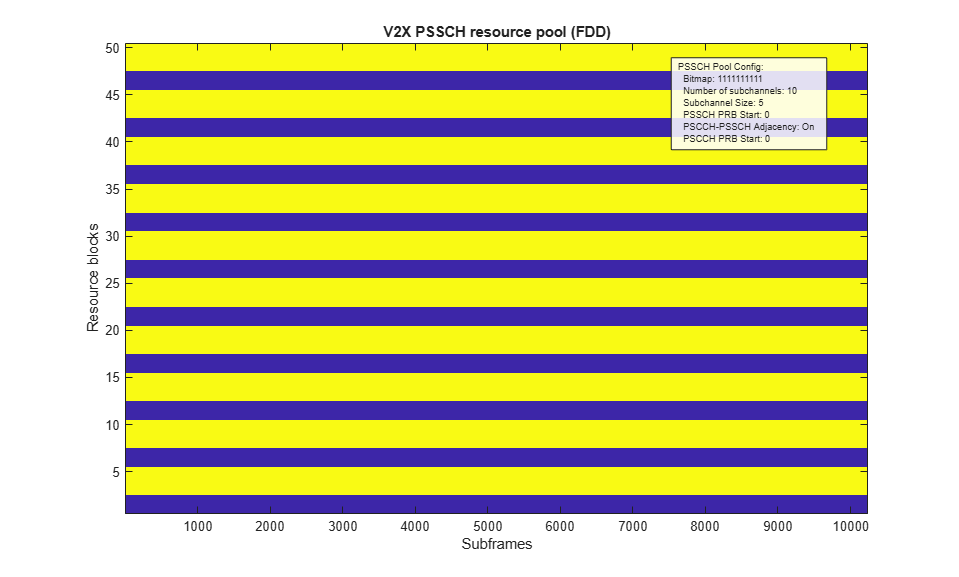### Processing Chain

To determine the throughput at each SNR point, the subframe by subframe processing chain includes:

• Update configuration for current HARQ process - The UE either carries new transport data or a retransmission of previously sent PSSCH transport data with a different redundancy version. All this is handled by the HARQ scheduler. The HARQ buffer `decState` stores the decoder state for soft combining.

• Create and encode the SCI message - The SCI message carries sidelink scheduling information. The SCI parameters are: `Priority` indicating ProSe Per-Packet Priority, `ResourceReservation` set by higher layers for reserving the resource for the next transmission of the transport block, `RIV` carrying allocation information, `TimeGap` signaling the time gap in subframes between the initial transmission and retransmission, `ModCoding` signaling the modulation scheme are used for PSSCH reception and `RetransmissionIdx` indicating whether the transmission is the first or second (retransmission). The SCI message is then encoded and mapped on to the PSCCH for transmission. The CRC of the SCI message is the scrambling identity for PSSCH.

• Plot the allocated resources - The resource allocation for the first HARQ process is plotted if the `displaySimulationInformation` flag is enabled. All HARQ processes have the same PSSCH (orange) and PSCCH (green) resource block allocation. The PSCCH and PSSCH can be transmitted on adjacent or non-adjacent resource blocks.

• Create Transmit Waveform - Pass the data generated by the UE to the physical layer processing stage to produce an SC-FDMA modulated waveform, containing the PSCCH and PSSCH physical channels and DRS signals.

• Noisy Channel Modeling - Pass the waveform through a fading channel and add noise (AWGN).

• Perform Blind Detection of PSCCH - The PSCCH resources and cyclic shift used in the transmitter in the subframe is unknown, so the synchronization, channel estimation and decoding is performed for each PRB set in the PSCCH resource block pool (provided by resourcePool.PSCCHResourceBlockPool) for all cyclic shifts until the SCI is successfully decoded or the entire resource pool is searched. The timing offset for the DM-RS correlation with the strongest peak is used for synchronization.

• Perform PSCCH channel estimation: PSCCH channel estimation is performed using lteSLChannelEstimatePSCCH. The channel estimator also produces an estimate of the noise power which can be used for MMSE equalization.

• Extract the PSCCH symbols and channel estimate: The received PSCCH symbols are extracted from the subframe resource grid, the corresponding channel estimates are extracted using lteExtractResources and the indices provided by ltePSCCHIndices.

• Perform PSCCH equalization: The PSCCH symbols are MMSE equalized using lteEqualizeMMSE with the channel estimate and noise estimate obtained above.

• Perform PSCCH demodulation: The equalized PSCCH symbols are demodulated using ltePSCCHDecode. This function performs the inverse of the transmitter modulation steps (SC-FDMA transform deprecoding, QPSK symbol demodulation and descrambling).

• Perform SCI decoding: SCI decoding is attempted using lteSCIDecode. The number of original information bits in the SCI message is given by lteSCIInfo. If the decoded CRC is zero and the recovered CRC mask is the expected value, the SCI decoding is considered successful and the decoded message bits are converted into the corresponding message structure using lteSCI. The CRC mask value provides the PSSCH scrambling identity, `NXID`.

The behavior of the shared channel decoding with respect to a failed SCI decoding is controlled by the variable `sciAssumed`. If `sciAssumed` is false, the failed SCI decoding immediately implies a failure to decode the SL-SCH and no further processing takes place for the current subframe. If `sciAssumed` is true, the transmitted SCI message is assumed to be known to the receiver and will be used in place of the received SCI message. If `sciAssumed` is true or if SCI decoding was successful, the receiver proceeds with SL-SCH decoding. Note that if SCI BLER measurement is disabled but SL-SCH throughput measurement is enabled then the transmitted SCI message is assumed to be known to the receiver.

For SL-SCH decoding:

• Get the PSSCH resource allocation - The RIV obtained from the recovered SCI message is then decoded to get the number of subchannels and the starting sub channel allocated for this transmission. This in combination with the PSCCH adjacency, size of each subchannel and first allocated resource block provides the set of allocated PSSCH RBs `PRBSet`.

• Synchronize and SC-FDMA demodulate subframe carrying PSSCH: The appropriate subframe of the waveform is synchronized using lteSLFrameOffsetPSSCH. The synchronized waveform is SC-FDMA demodulated using lteSLSCFDMADemodulate. Note that although the control and shared channel are transmitted in the same subframe, it is sufficient to perform the synchronization and SC-FDMA demodulation only for the PSCCH. However, in this example, since the shared and control channel can be independently received, PSSCH synchronization and SC-FDMA demodulation are performed. This is to enable PSSCH reception when the PSCCH reception is disabled.

• Perform PSSCH channel estimation: PSSCH channel estimation is performed using lteSLChannelEstimatePSSCH. The channel estimator also produces an estimate of the noise power which can be used for MMSE equalization.

• Extract the PSSCH symbols and channel estimate: The received PSSCH symbols are extracted from the subframe resource grid and the corresponding channel estimates are extracted using lteExtractResources and the indices provided by ltePSSCHIndices.

• Perform PSSCH equalization: The PSSCH symbols are MMSE equalized using lteEqualizeMMSE with the channel estimate and noise estimate obtained above.

• Perform PSSCH demodulation: The equalized PSSCH symbols are demodulated using ltePSSCHDecode. This function performs the inverse of the transmitter modulation steps (SC-FDMA transform deprecoding, QPSK or 16QAM symbol demodulation and descrambling). The descrambling uses the PSCCH CRC obtained from the SCI decoding stage.

• Decoding the Sidelink Shared Channel (SL-SCH) and Storing the Block CRC Error for a UE - Pass the vector of decoded soft bits to lteSLSCHDecode, which decodes the codeword and returns the block CRC error used to determine the throughput of the system. The contents of the new soft buffer, `harqProcesses(harqID).decState`, is available at the output of this function to be used when decoding the next subframe.

```% Initialize variables used in the simulation and analysis maxThroughputSLSCH = zeros(length(SNRIn),1); simThroughputSLSCH = zeros(length(SNRIn),1); simBLERSCI = zeros(length(SNRIn),1); % Get the number of HARQ processes required nHARQProcesses = floor(sfGap/tti); % Calculate the HARQ ID sequence for each of the transmitting subframes harqProcessSequence = zeros(1,NFrames*10); for h = 1:nHARQProcesses harqProcessSequence(1+tti*(h-1):sfGap:NFrames*10) = h; end % Create the partial SCI message with the parameters that have fixed values % for the simulation. Other parameters that have variable values will be % set further down in the simulation loop sciMessage = struct('SCIFormat','Format1'); sciMessage.TimeGap = sfGap; % Set the PSSCH MCS according to the TBS and modulation scheme [itbs,modn] = lteMCS(0:28,'PUSCH'); % According to TS 36.213 Section 14.1.1, for IMCS (0 to 28), the modulation % order is set to Q' = min(4,Qm') where Qm' can be 2, 4 or 6. So change the % cases where Qm' = 6 ('64QAM') to Qm' = 4 ('16QAM') modn(strcmpi(modn, '64QAM')) = {'16QAM'}; % Filter valid transport block sizes according to modulation scheme used possibleTBS = lteTBS(NPRB,itbs); possibleTBS(~strcmpi(modn,ueConfig.Modulation)) = 0; % Now set the MCS index according to the TBS sciMessage.ModCoding = find(possibleTBS==TBS,1) - 1; % Create new figure for displaying resource allocation, if required if displaySimulationInformation figure; end % If SCI BLER is not being measured, 'sciAssumed' must be set so that the % receiver can assume knowledge of the SCI if (~measureBLERForSCI) sciAssumed = true; end rng('default'); for snrIdx = 1:numel(SNRIn) % Set the random number generator seed depending on the loop variable % to ensure independent random streams rng(snrIdx); % Initialize the state of all HARQ buffers harqProcess = struct('RVIdx',1,'data',[],'decState',[]); harqProcesses(1:nHARQProcesses) = harqProcess; RVSeq = [0 2]; % RV for initial transmission and retransmission % Set up variables for the main loop lastOffset = 0; % Initialize overall frame timing offset frameOffset = 0; % Initialize frame timing offset sharedbitTput = []; % Number of successfully received bits per subframe txedTrBlkSizes = []; % Number of transmitted bits per subframe controlErrors = []; % Number of SCI block errors for subframeNo = 0:(NFrames*10-1) harqID = harqProcessSequence(subframeNo+1); if harqID == 0 % If there is no HARQ process transmitting in the current % subframe, continue to the next continue end % Update current HARQ process with new transport data and reset the % receive buffer if it is the initial transmission. The PSSCH is % transmitted twice with a gap of 'sfGap' subframes irrespective of % the success of the first transmission if harqProcesses(harqID).RVIdx == 1 harqProcesses(harqID).data = randi([0 1], TBS, 1); harqProcesses(harqID).decState = []; end % Set the RV ueConfig.RV = RVSeq(harqProcesses(harqID).RVIdx); % Set the subframe number ueConfig.NSubframePSSCH = subframeNo; % Display run time information if displaySimulationInformation fprintf('Subframe: %d. HARQ process index: %d. Redundancy version: %d\n',subframeNo,harqID,ueConfig.RV) end % Channel time for the current subframe channel.InitTime = subframeNo/1000; % create an empty resource grid slgrid = lteSLResourceGrid(ueConfig); % Update the transmission config with the starting RB startingRB = rbStart(harqProcesses(harqID).RVIdx); resourcePool.Config.startRB_Subchannel_r14 = startingRB; % Calculate the RIV from the number of allocated subchannels and % the starting subchannel ueConfig.FirstSubchannelIdx = 0; beta = 0; if strcmpi(resourcePool.Config.adjacencyPSCCH_PSSCH_r14,'On') beta = 2; end lsubCH = ceil((NPRB+beta)/resourcePool.Config.sizeSubchannel_r14); sciMessage.RIV = resourcePool.encodeRIV(lsubCH,ueConfig.FirstSubchannelIdx); % Set the retransmission index sciMessage.RetransmissionIdx = harqProcesses(harqID).RVIdx - 1; % Create the SCI and message bits sciConfig = ueConfig; sciConfig.PSSCHNSubchannels = resourcePool.Config.numSubchannel_r14; [sciMessage, sciBits] = lteSCI(sciConfig,sciMessage); % Perform SCI encoding [cw,crc] = lteSCIEncode(sciConfig, sciBits); % NXID is the 16 bit CRC associated with the PSCCH SCI grant and is % used as the PSSCH scrambling identity ueConfig.NXID = crc; % Store NXID value in 'expectedNXID' so that the receiver won't % have to access 'sciMessage' at all expectedNXID = ueConfig.NXID; % Display the subframes and resource blocks for transmission. Note % that the allocations are the same for all HARQ processes and that % the plot does not show the subframe offset for HARQ processes % other than 1 if (displaySimulationInformation) && (harqID==1) % Specify the additional parameters required for the resource % pool display sciMessage.FirstSubchannelIdx = ueConfig.FirstSubchannelIdx; sciMessage.SLIndex = 0; resourcePool.displayPeriod(sciMessage,10); drawnow; end % Create the PSCCH codeword pscchSymbols = ltePSCCH(cw); % Get the resource allocation for the PSCCH [pscchsf,pscchrb] = resourcePool.getPSCCHResources(setfield(sciMessage,'FirstSubchannelIdx',ueConfig.FirstSubchannelIdx)); %#ok<SFLD> % Select the resource for this transmisison sciConfig.PRBSet = pscchrb(:,harqProcesses(harqID).RVIdx); % Get the PSCCH symbol indices pscchIndices = ltePSCCHIndices(sciConfig); % Map the PSCCH symbols on to the grid slgrid(pscchIndices) = pscchSymbols; % Define a random cyclic shift from the set {0,3,6,9} for each % PSCCH transmission sciConfig.CyclicShift = randi([0 3])*3; % Create the DM-RS symbols and indices pscchdrssymbols = ltePSCCHDRS(sciConfig); pscchdrsindices = ltePSCCHDRSIndices(sciConfig); % Map the PSCCH DRS symbols on to the grid slgrid(pscchdrsindices) = pscchdrssymbols; % Get the resource allocations for the two transmissions [psschsf,psschrb] = resourcePool.getPSSCHResources(setfield(sciMessage,'FirstSubchannelIdx',ueConfig.FirstSubchannelIdx)); %#ok<SFLD> % Now select the resource for this transmission ueConfig.PRBSet = psschrb(:,harqProcesses(harqID).RVIdx); % Calculate the PSSCH resource indices [psschIndices,psschinfo] = ltePSSCHIndices(ueConfig); % SL-SCH and PSSCH cw = lteSLSCH(ueConfig,psschinfo.G,harqProcesses(harqID).data); psschSymbols = ltePSSCH(ueConfig,cw); slgrid(psschIndices) = psschSymbols; % PSSCH DRS and DRS indices psschdrssymbols = ltePSSCHDRS(ueConfig); psschdrsindices = ltePSSCHDRSIndices(ueConfig); slgrid(psschdrsindices) = psschdrssymbols; % The SC-FDMA modulation will not use windowing due to the % piece-wise nature of the waveform construction windowing = 0; % perform sidelink SC-FDMA modulation [txWaveform,scfdmaInfo] = lteSLSCFDMAModulate(ueConfig,slgrid,windowing); % Add 25 sample padding. This is to cover the range of delays % expected from channel modeling (a combination of % implementation delay and channel delay spread) ntxants = size(txWaveform,2); txWaveform = [txWaveform; zeros(25, ntxants)]; %#ok<AGROW> % Pass data through channel model rxNoiselessWaveform = lteFadingChannel(channel,txWaveform); % Calculate noise gain SNR = 10^((SNRIn(snrIdx))/20); % Normalize noise power to take account of sampling rate, % which is a function of the IFFT size used in OFDM % modulation, and the number of antennas N0 = 1/(sqrt(2.0*ntxants*double(ofdmInfo.Nfft))*SNR); % Create AWGN noise = N0*complex(randn(size(rxNoiselessWaveform)),... randn(size(rxNoiselessWaveform))); % Add AWGN to the received time domain waveform rxWaveform = rxNoiselessWaveform + noise; %------------------------------------------------------------------ % Receiver %------------------------------------------------------------------ % Define the receiver top-level configuration rxConfig = []; rxConfig.SidelinkMode = ueConfig.SidelinkMode; rxConfig.CyclicPrefixSL = ueConfig.CyclicPrefixSL; rxConfig.FirstSubchannelIdx = ueConfig.FirstSubchannelIdx; rxConfig.NSLRB = resourcePool.Config.NSLRB; rxConfig.DuplexMode = resourcePool.Config.DuplexMode; rxConfig.TDDConfig = resourcePool.Config.TDDConfig; rxConfig.PSSCHNSubchannels = resourcePool.Config.numSubchannel_r14; rxConfig.PSSCHSubchannelSize = resourcePool.Config.sizeSubchannel_r14; rxConfig.PSSCHAdjacency = resourcePool.Config.adjacencyPSCCH_PSSCH_r14; rxConfig.PSSCHSubchannelPRBStart = resourcePool.Config.startRB_Subchannel_r14; rxConfig.NSubframePSSCH = subframeNo; % Set the subframe number % If SCI BLER measurement is configured: sciDecoded = false; if measureBLERForSCI % Blind decoding of the control channel % We know that the current subframe contains control and data, % but do not know the location. Check for the SCI message in % the resource pool for all cyclic shifts till it is found or % the whole resource pool is searched pscchPool = resourcePool.PSCCHResourceBlockPool; % Re-arrange the resources into the possible PRB pairs pscchPool = reshape(pscchPool,2,numel(pscchPool)/2); p = 1; while p <= size(pscchPool,2) && ~sciDecoded % PSCCH DRS for V2X is transmitted on two consecutive RBs rxConfig.PRBSet = pscchPool(:,p); for cs = [0 3 6 9] rxConfig.CyclicShift = cs; [frameOffset,corr] = lteSLFrameOffsetPSCCH(rxConfig,rxWaveform); % Perform subframe synchronization if (frameOffset > 25) || (frameOffset < 0) frameOffset = lastOffset; end lastOffset = frameOffset; % Perform SC-FDMA demodulation on the received data to obtain % the resource grid rxSubframe = lteSLSCFDMADemodulate(rxConfig,rxWaveform(1+frameOffset:end,:)); % Perform channel estimation if(perfectChanEstimator) [hest,nest] = perfectChannelEstimate(rxConfig,channel,noise,frameOffset); else [hest,nest] = lteSLChannelEstimatePSCCH(rxConfig,cec,rxSubframe); end % Get the PSCCH candidate for the current PRBSet, % extract the corresponding received symbols and % channel estimate and perform equalization pscchCandidate = ltePSCCHIndices(rxConfig); [pscchRx,pscchHest] = lteExtractResources(pscchCandidate,rxSubframe,hest); pscchSymbols = lteEqualizeMMSE(pscchRx,pscchHest,nest); % Demodulate the PSCCH codedsciBits = ltePSCCHDecode(pscchSymbols); % Get the payload size for the Release 14 V2X SCI message scilengthinfo = lteSCIInfo(rxConfig); % Decode the SCI and recover the message [rxinfo,rxSCIErr,rxcrc] = lteSCIDecode(scilengthinfo.Format1,codedsciBits); if ~rxSCIErr && (rxcrc==expectedNXID) sciMessageRx = lteSCI(rxConfig,rxinfo); rxConfig.NXID = rxcrc; sciDecoded = true; if (displaySimulationInformation) fprintf(' SCI decoded\n'); end break; end end % Increment the pool index p = p+1; end if ~sciDecoded if (displaySimulationInformation) fprintf(' SCI decoding failed\n'); end end % Store values needed to calculate BLER controlErrors = [controlErrors ~sciDecoded]; %#ok<AGROW> end % If SCI decoding failed and the SCI is assumed for SL-SCH % decoding, set the decoded SCI message equal to the transmitted % SCI message. Also set the NXID to the expected value if (~sciDecoded) && (sciAssumed) sciMessageRx = sciMessage; rxConfig.NXID = expectedNXID; end % If SL-SCH throughput measurement is configured and if the SCI was % successfully decoded or if SCI decoding success is assumed, % perform PSSCH reception and SL-SCH decoding if measureTputForSLSCH if (sciDecoded || sciAssumed) % Get the PSSCH resource allocation from the decoded SCI % message and pool configuration rxConfig.PRBSet = lteSCIResourceAllocation(rxConfig,sciMessageRx); % Perform subframe synchronization frameOffset = lteSLFrameOffsetPSSCH(rxConfig,rxWaveform); if (frameOffset > 25) || (frameOffset < 0) frameOffset = lastOffset; end lastOffset = frameOffset; % Perform SC-FDMA demodulation on the received data to obtain % the resource grid rxSubframe = lteSLSCFDMADemodulate(rxConfig,rxWaveform(1+frameOffset:end,:)); % Perform channel estimation, extract the received PSSCH % symbols and the corresponding channel estimate, and % perform equalization if(perfectChanEstimator) [hest,nest] = perfectChannelEstimate(rxConfig,channel,noise,frameOffset); else [hest,nest] = lteSLChannelEstimatePSSCH(rxConfig,cec,rxSubframe); end [psschRx,psschHest] = lteExtractResources(psschIndices,rxSubframe,hest); psschSymbols = lteEqualizeMMSE(psschRx,psschHest,nest); % Demodulate the PSSCH [~,rxConfig.Modulation] = lteMCS(sciMessageRx.ModCoding,'PUSCH'); if (strcmpi(rxConfig.Modulation,'64QAM')) rxConfig.Modulation = '16QAM'; end codedSlschBits = ltePSSCHDecode(rxConfig,psschSymbols); % Decode the SL-SCH, including soft combining into the % receiver buffer and check the CRC rxConfig.NTurboDecIts = 5; % Turbo decoder iterations rxConfig.RV = RVSeq(harqProcesses(harqID).RVIdx); [~,slschCRC,harqProcesses(harqID).decState] = ... lteSLSCHDecode(rxConfig,TBS,codedSlschBits, ... harqProcesses(harqID).decState); slschDecoded = ~slschCRC; else % If SCI decoding failed, SL-SCH decoding also failed slschDecoded = false; end % Store values needed to calculate throughput sharedbitTput = [sharedbitTput TBS*slschDecoded]; %#ok<AGROW> txedTrBlkSizes = [txedTrBlkSizes TBS]; %#ok<AGROW> if (displaySimulationInformation) if slschDecoded fprintf(' SL-SCH decoded\n'); else fprintf(' SL-SCH decoding failed\n'); end end end % Update the RV sequence index for the next transmission harqProcesses(harqID).RVIdx = mod(harqProcesses(harqID).RVIdx,size(RVSeq,2))+1; end if measureBLERForSCI % Calculate the SCI BLER simBLERSCI(snrIdx) = 100*mean(controlErrors,2); % Display the results dynamically in the command window fprintf('\nSNR = %.2f dB. SCI BLER(%%) for %d Frame(s) = %.4f %%\n',... SNRIn(snrIdx),NFrames,simBLERSCI(snrIdx)); end if measureTputForSLSCH % Calculate the maximum and simulated throughput maxThroughputSLSCH(snrIdx) = sum(txedTrBlkSizes); % Max possible throughput simThroughputSLSCH(snrIdx) = sum(sharedbitTput); % Simulated throughput % Display the results dynamically in the command window fprintf('\nSNR = %.2f dB. SL-SCH Throughput for %d Frame(s) = %.4f Mbps\n',... SNRIn(snrIdx),NFrames,1e-6*simThroughputSLSCH(snrIdx)/(NFrames*10e-3)); fprintf('SNR = %.2f dB. SL-SCH Throughput(%%) for %d Frame(s) = %.4f %%\n',... SNRIn(snrIdx),NFrames,simThroughputSLSCH(snrIdx)*100/maxThroughputSLSCH(snrIdx)); end end ```
```Subframe: 0. HARQ process index: 1. Redundancy version: 0 SCI decoding failed SL-SCH decoding failed Subframe: 4. HARQ process index: 2. Redundancy version: 0 SCI decoded SL-SCH decoding failed Subframe: 8. HARQ process index: 1. Redundancy version: 2 SCI decoded SL-SCH decoding failed Subframe: 12. HARQ process index: 2. Redundancy version: 2 SCI decoded SL-SCH decoded Subframe: 16. HARQ process index: 1. Redundancy version: 0 SCI decoded SL-SCH decoding failed Subframe: 20. HARQ process index: 2. Redundancy version: 0 SCI decoded SL-SCH decoding failed Subframe: 24. HARQ process index: 1. Redundancy version: 2 SCI decoded SL-SCH decoded Subframe: 28. HARQ process index: 2. Redundancy version: 2 SCI decoded SL-SCH decoded Subframe: 32. HARQ process index: 1. Redundancy version: 0 SCI decoded SL-SCH decoding failed Subframe: 36. HARQ process index: 2. Redundancy version: 0 SCI decoded SL-SCH decoding failed SNR = -5.00 dB. SCI BLER(%) for 4 Frame(s) = 10.0000 % SNR = -5.00 dB. SL-SCH Throughput for 4 Frame(s) = 0.2622 Mbps SNR = -5.00 dB. SL-SCH Throughput(%) for 4 Frame(s) = 30.0000 % Subframe: 0. HARQ process index: 1. Redundancy version: 0 SCI decoded SL-SCH decoded Subframe: 4. HARQ process index: 2. Redundancy version: 0 SCI decoded SL-SCH decoded Subframe: 8. HARQ process index: 1. Redundancy version: 2 SCI decoded SL-SCH decoded Subframe: 12. HARQ process index: 2. Redundancy version: 2 SCI decoded SL-SCH decoded Subframe: 16. HARQ process index: 1. Redundancy version: 0 SCI decoded SL-SCH decoded Subframe: 20. HARQ process index: 2. Redundancy version: 0 SCI decoded SL-SCH decoded Subframe: 24. HARQ process index: 1. Redundancy version: 2 SCI decoded SL-SCH decoded Subframe: 28. HARQ process index: 2. Redundancy version: 2 SCI decoded SL-SCH decoded Subframe: 32. HARQ process index: 1. Redundancy version: 0 SCI decoded SL-SCH decoded Subframe: 36. HARQ process index: 2. Redundancy version: 0 SCI decoded SL-SCH decoded SNR = 0.00 dB. SCI BLER(%) for 4 Frame(s) = 0.0000 % SNR = 0.00 dB. SL-SCH Throughput for 4 Frame(s) = 0.8740 Mbps SNR = 0.00 dB. SL-SCH Throughput(%) for 4 Frame(s) = 100.0000 % Subframe: 0. HARQ process index: 1. Redundancy version: 0 SCI decoded SL-SCH decoded Subframe: 4. HARQ process index: 2. Redundancy version: 0 SCI decoded SL-SCH decoded Subframe: 8. HARQ process index: 1. Redundancy version: 2 SCI decoded SL-SCH decoded Subframe: 12. HARQ process index: 2. Redundancy version: 2 SCI decoded SL-SCH decoded Subframe: 16. HARQ process index: 1. Redundancy version: 0 SCI decoded SL-SCH decoded Subframe: 20. HARQ process index: 2. Redundancy version: 0 SCI decoded SL-SCH decoded Subframe: 24. HARQ process index: 1. Redundancy version: 2 SCI decoded SL-SCH decoded Subframe: 28. HARQ process index: 2. Redundancy version: 2 SCI decoded SL-SCH decoded Subframe: 32. HARQ process index: 1. Redundancy version: 0 SCI decoded SL-SCH decoded Subframe: 36. HARQ process index: 2. Redundancy version: 0 SCI decoded SL-SCH decoded SNR = 5.00 dB. SCI BLER(%) for 4 Frame(s) = 0.0000 % SNR = 5.00 dB. SL-SCH Throughput for 4 Frame(s) = 0.8740 Mbps SNR = 5.00 dB. SL-SCH Throughput(%) for 4 Frame(s) = 100.0000 % ```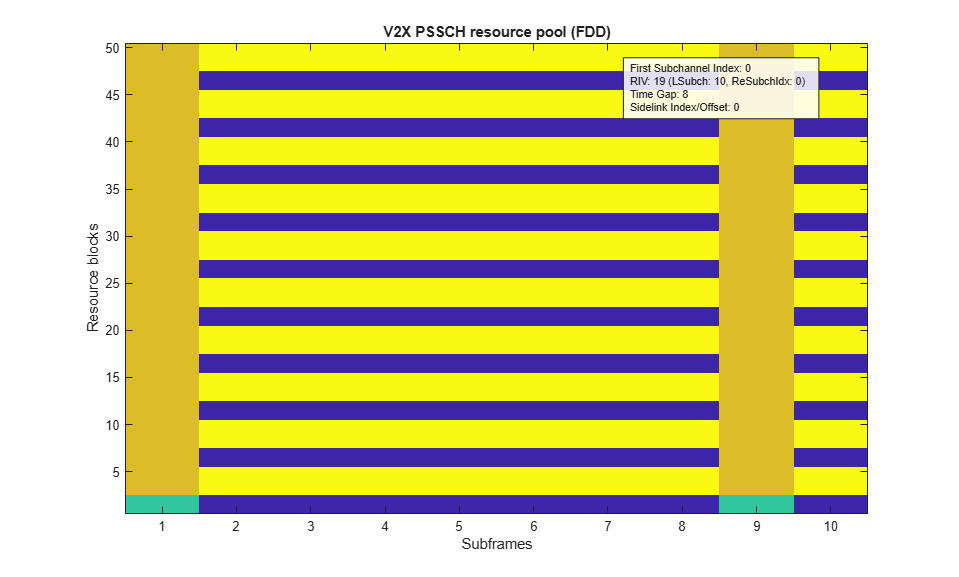### Throughput Results

The simulation results are displayed in the MATLAB® command window after each SNR point is completed. They are also captured in output arrays `simBLERSCI`, `simThroughputSLSCH` and `maxThroughputSLSCH`. For each simulated SNR point, `simBLERSCI` stores the measured SCI BLER, `simThroughputSLSCH` stores the measured SL-SCH throughput in number of bits and `maxThroughputSLSCH` stores the maximum possible SL-SCH throughput in number of bits.

```if measureBLERForSCI % Plot PSCCH/SCI BLER plotResults('SCI', 'BLER',channel,SNRIn,simBLERSCI); end if measureTputForSLSCH % Plot PSSCH/SL-SCH throughput plotResults('SL-SCH', 'Throughput',channel,SNRIn,simThroughputSLSCH*100./maxThroughputSLSCH); end ```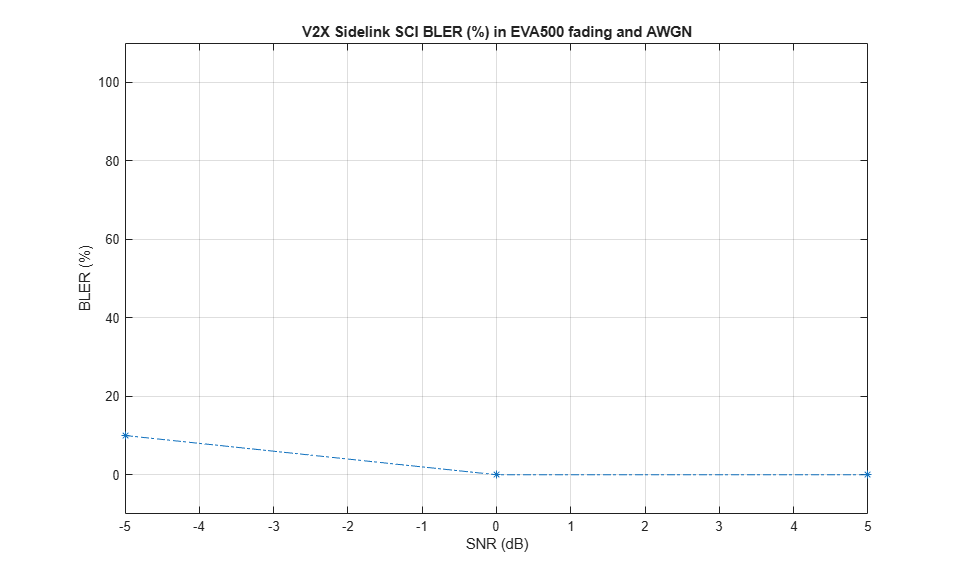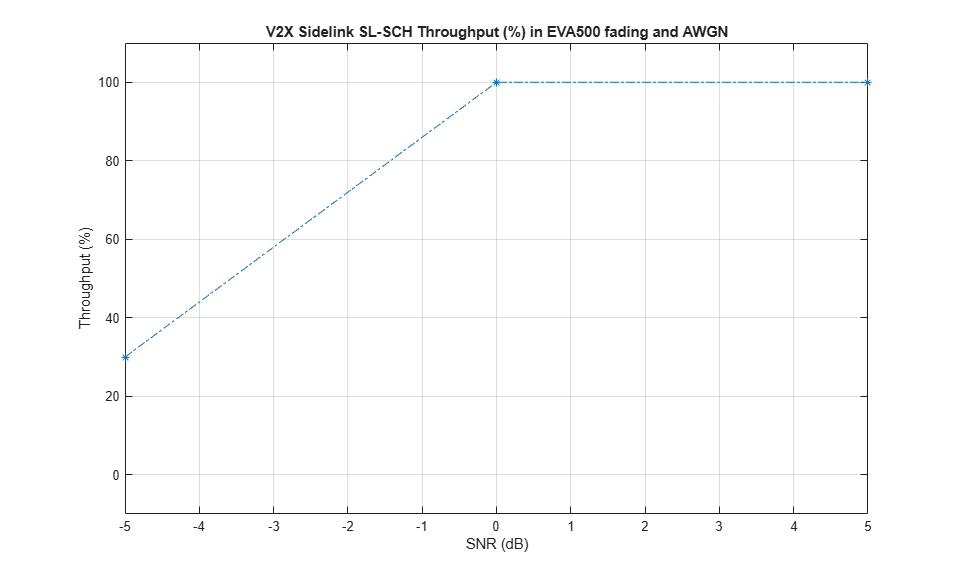For statistically valid results, the simulation should be run for a larger number of frames. The figures below show the SCI BLER and SL-SCH throughput results when simulating 1000 frames for an extended range of SNR values with practical channel estimation.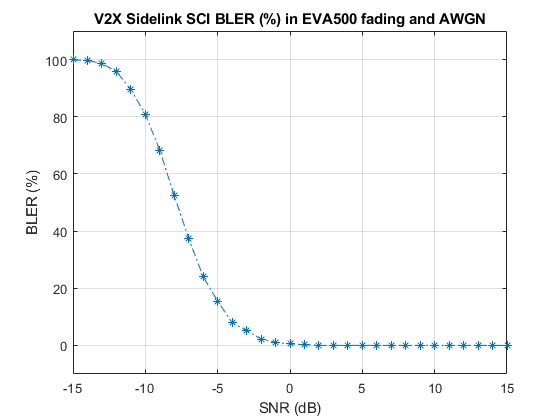### Selected Bibliography

1. 3GPP TS 36.213 "Physical layer procedures"

2. 3GPP TS 36.101 "User Equipment (UE) radio transmission and reception"

### Local Functions

The following local functions are used in this example:

• `perfectChannelEstimate`: perfect channel estimation

• `plotResults`: plot the results of the example

```% Calculate the perfect channel estimate and the noise estimate function [hest,nest] = perfectChannelEstimate(rxConfig,channel,noise,frameOffset) % Use the uplink perfect channel estimator to calculate % the sidelink estimate as both use SC-FDMA rxConfig.NTxAnts = 1; rxConfig.NULRB = rxConfig.NSLRB; hest = lteULPerfectChannelEstimate(rxConfig,channel,frameOffset); noiseGrid = lteSLSCFDMADemodulate(rxConfig,noise(1+frameOffset:end ,:)); nest = var(noiseGrid(:)); end % Plot the SCI BLER or SL-SCH Throughput function plotResults(chName,simOpt,channel,xvalues,yvalues) fadingDescription = sprintf('%s%s',channel.DelayProfile,num2str(channel.DopplerFreq)); figure; plot(xvalues, yvalues,'*-.'); v = axis; axis([v(1) v(2) -10 110]) title(sprintf('V2X Sidelink %s %s (%%) in %s fading and AWGN',chName,simOpt,fadingDescription)); xlabel('SNR (dB)'); ylabel([simOpt ' (%)']); grid on; end ```